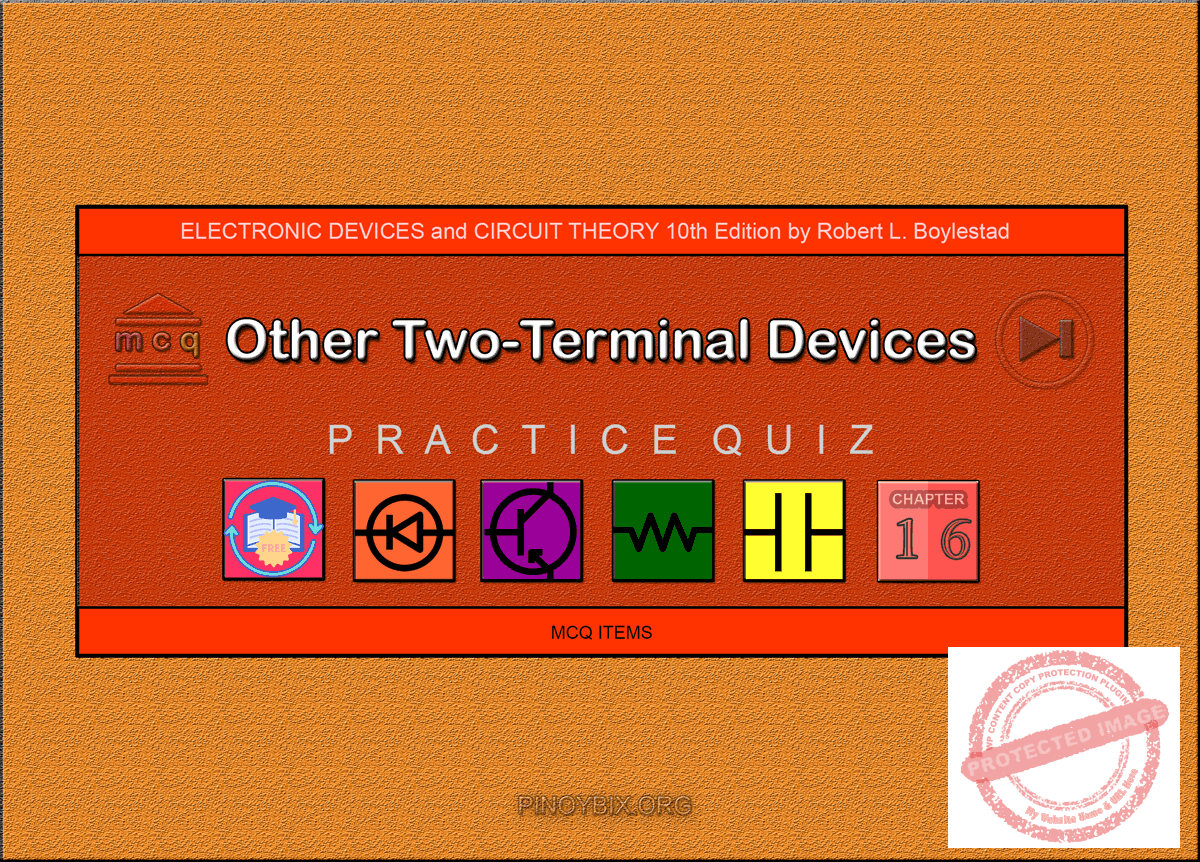(Last Updated On: February 4, 2020)Below are the answers key for the Multiple Choice Questions (Quiz) in Other Two-Terminal Devices from the book Electronic Devices and Circuit Theory 10th Edition by Robert L. Boylestad.

1. D. All of the above

2. D. All of the above

3. C. Microamperes, milliamperes

4. A. 0 V to 0.2 V

5. E. Any of the above

6. B 50

7. A. 20 GHz

8. A. Schottky

9. D. 2 pF to 100 pF

10. D. All of the above

11. C. voltage, capacitor

12. B. varicap

13. C. exponentially

14. D. silicon

15. B. False

16. A. True

17. C. Tunnel

18. C. Both germanium and gallium arsenide

19. D. 20:1

20. A. A few microamperes to several hundred amperes

21. D. 600 mV

22. B. Positive-resistance

23. C. Photodiode

24. A. 100 ms

25. D. All of the above

26. C. 60°C

27. A. Less than 100 ns

28. C. 100 ms to 300 ms

29. B. 100 mW/cm2

30. D. All of the above

31. C. Either positive or negative

32. D. All of the above

33. A. 5 kΩ

34. C. 100 Ω

35. B. 3% to 5%

#### FILL-IN-THE-BLANKS

1. C. both quick response time and a lower noise figure

2. A. more, high

3. B. electron

4. C. less than

5. B. a higher

6. B. 1/3

7. D. All of the above

8. C. increases, reduces

9. B. 20 V

10. A. very large, very small

11. D. All of the above

12. C. parallel, series

13. B. decreases

14. D. one hundred, several thousand

15. D. All of the above

16. C. both angstrom units and micrometers

17. A. increases

18. B. higher, higher

19. D. 10 ms

20. B. increases

21. A. a lower

22. B. much slower than

23. C. Both selenium and silicon

24. D. All of the above

25. A. 10%, 40%